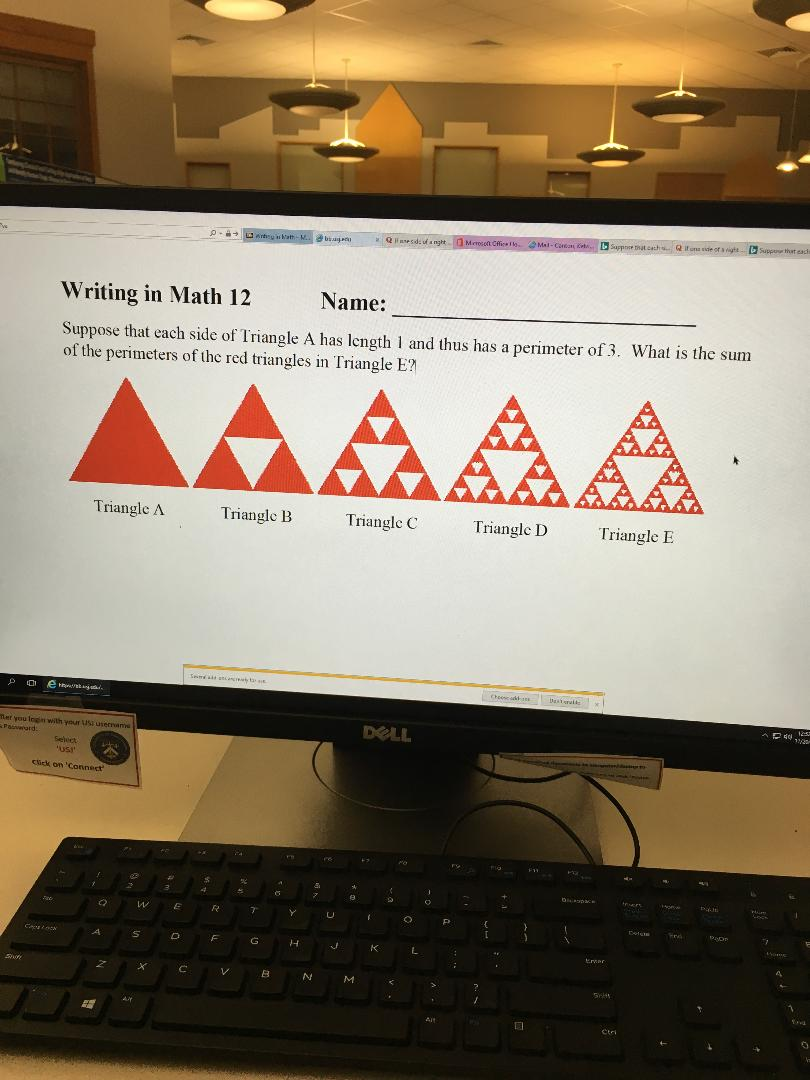# Suppthat cachbSuppe that eachsQifons side of s ighsSuppe atcachMal-Coten tMeresot Cfice Ianhy s ath-MQaesdefangtbName:Writing in Math 12Suppose that each side of Triangle A has length 1 and thus has a perimeter of 3. What is the sumof the perimeters of the red triangles in Triangle E?AAAAATriangle ATriangle BTriangle CTriangle DTriangle ECheese a sesa1/amDELLer you legis with your US usernatiPa dSelectusImSck on 'Connect424WERYUOPetersAFGHLcrarAXCVMSeAYAitCtri

Question
32 views

anyone good with traingles?help_outlineImage TranscriptioncloseSupp that cach bSuppe that eachs Qifons side of s ighs Suppe atcach Mal-Coten t Meresot Cfice I anhy s ath-M Qaesdefangt b Name: Writing in Math 12 Suppose that each side of Triangle A has length 1 and thus has a perimeter of 3. What is the sum of the perimeters of the red triangles in Triangle E? AAAAA Triangle A Triangle B Triangle C Triangle D Triangle E Cheese a s esa 1/am DELL er you legis with your US usernati Pa d Select usI m Sck on 'Connect 4 2 4 W E R Y U O P eters A F G H L crar A X C V M Se AY Ait Ctri fullscreen
check_circle

Step 1

The perimeter of a triangle is the sum of all the sides of triangles.

Length of each side of triangle A = 1.

And since the perimeter of triangle A is given 3 therefore, we can conclude that the triangle is an equilateral triangle.

Let M = 3.

Step 2

Now in triangle B, the smaller triangle is made by joining the midpoints of the sides of the triangle A. Therefore, the smaller triangle is also an equilateral triangle and the length of sides of the smaller triangle will be half of the sides of the triangle A i.e 1/2. Therefore, the perimeter of smaller triangle in B is 3 × 1/2 = 3/2.

Let this value be N. Therefore, N = 3/2.

Step 3

Now in triangle C, the 3 smaller triangles are made by joining the midpoints of the triangles with the length of side ½. Therefore, the length of the sides of the 3 smaller triangles will be ¼. Now perimeter of one smaller triangle will be 3...

### Want to see the full answer?

See Solution

#### Want to see this answer and more?

Solutions are written by subject experts who are available 24/7. Questions are typically answered within 1 hour.*

See Solution
*Response times may vary by subject and question.
Tagged in

### Algebra